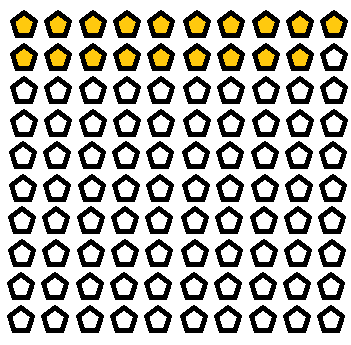# Percentages: Lesson for Kids

Instructions:

question 1 of 3

### What does the word percentage mean?

Create Your Account To Take This Quiz

As a member, you'll also get unlimited access to over 79,000 lessons in math, English, science, history, and more. Plus, get practice tests, quizzes, and personalized coaching to help you succeed.

Try it risk-free for 30 days. Cancel anytime

### 1. What percentage of the pentagons are colored in orange? There are 100 pentagons total.### 2. Which of the following is NOT equal to 10%?

Create your account to access this entire worksheet
Quizzes, practice exams & worksheets
Certificate of Completion
Create an account to get started

If you want to see what you know about percentages, you can use this quiz and worksheet. The quiz has multiple-choice questions on it, which help make learning fun.

The quiz and worksheet help you see how much you know about percentages. You will be quizzed on fractions and shown examples of percentages.

## Quiz & Worksheet Goals

Topics on the quiz include:

• Finding the sale price
• Fractions
• What percentage means
• Examples of different percentages

## Skills Practiced

If you use the quiz and worksheet, you can practice these skills:

• Information recall - remember the information you learned about using fractions and percentages
• Reading comprehension - pick out the most important information from the math lesson on percentages
• Knowledge application - use your knowledge to calculate sales prices

If you want to keep learning, use the lesson called, Percentages: Lesson for Kids. Use this lesson in order to:

• Learn the steps necessary to transform 50% into a decimal
• Write percentages as fractions
• Discover three ways of showing the same value
Final Exam
Algebra for Kids
This course currently has no practice test
Chapter ExamPercents for Elementary School
Status: Not Started

Support